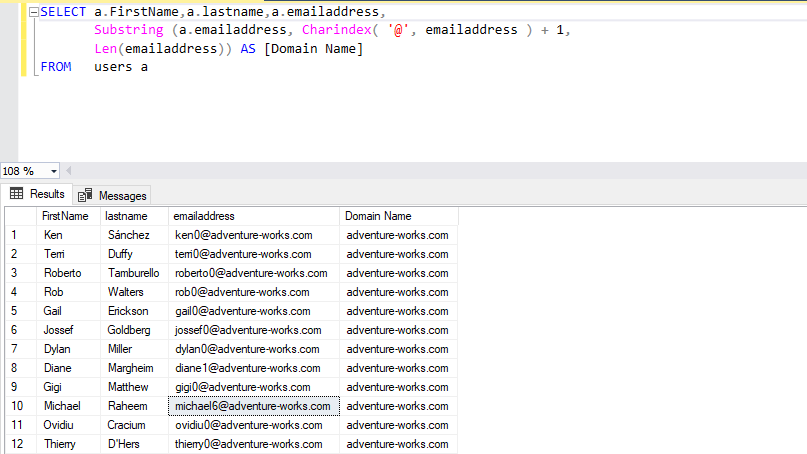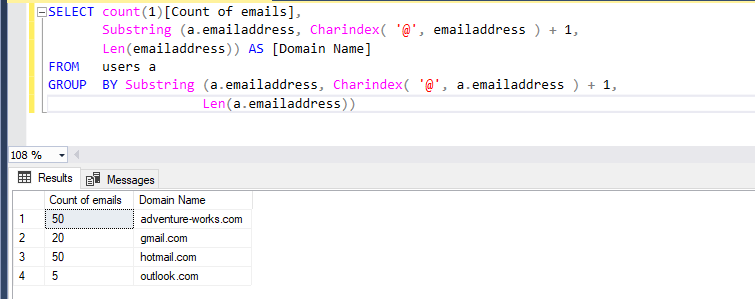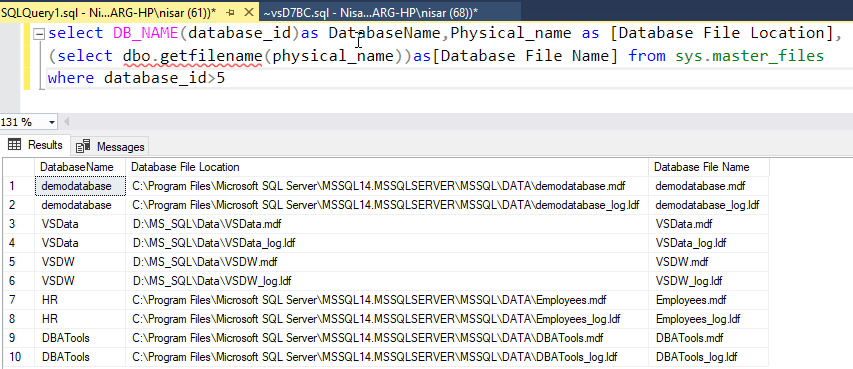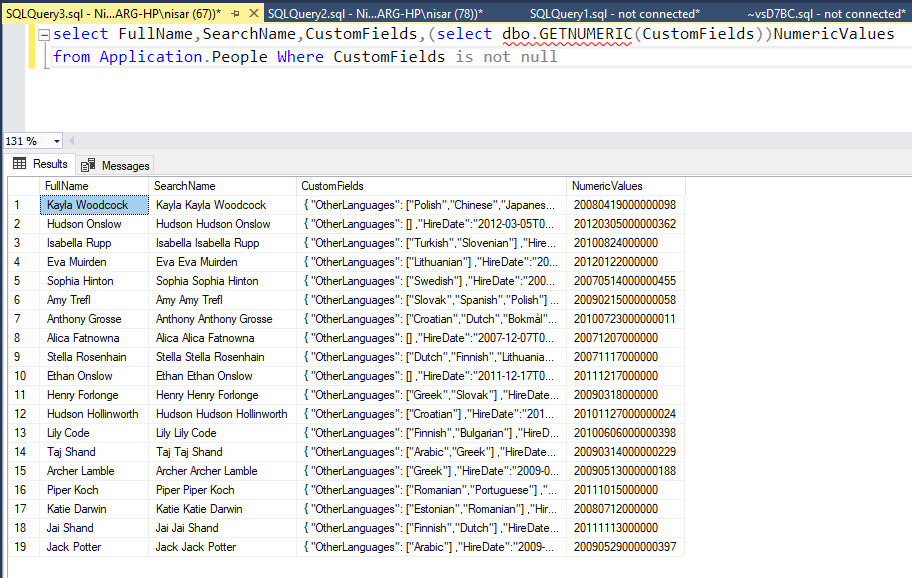# T-SQL Regular Expressions: SUBSTRING, PATINDEX and CHARINDEX

Total: 8 Average: 4.3

In my previous article about T-SQL regular expressions, I have explained the LIKE operator, its usage and provided several examples with it. In this article, we are going to discuss the SUBSTRING, PATINDEX, and CHARINDEX functions of T-SQL. These functions can be used to perform pattern matching.

First, let me explain the SUBSTRING function and provide examples with it.

### SUBSTRING

SQL Server SUBSTRING() function is used to extract the substring from the given input_string. It extracts the substring, starting from the specified position defined by the parameter.
Following is the syntax for the SUBSTRING()

`SUBSTRING(input_string, starting_position, length)`

SUBSTRING() function accepts following parameters:

1. Input_string. This parameter defines a string expression from which you want to extract the substring. This parameter can be text, character, or binary string.
2. Starting_position. This parameter defines a starting position from where you want to extract the substring. This parameter must be an integer value. You can use only one starting position parameter.
3. Length. This parameter defines a position of the character you want to extract from the input string. This parameter must be an integer value.

The following are the examples of the SUBSTRING function.

#### Example 1: Use SUBSTRING using literal string input

For example, I want to extract the word “DBA” from the input string “Nisarg is DBA.” In an input string, the position of the character D is eleven, and we want to extract three characters, therefore, the value of the “starting_position” parameter is 11, and the value of the “length” parameter is 3.

To do that, execute the following:

`SELECT Substring('Nisarg is DBA', 11, 3)`

Following is the output:

```Substring
---------
DBA```

#### Example 2: Use SUBSTRING within T-SQL Query

I have created a sample table named “IPDREGISTRATION” in the VSData database. In the table, there is a column named “IPDREGNO,” which represents patients’ registration number. The IPDREGNO is a unique number and its format is <IP> <Financial_Year><6 digit IncrementalNumber>. For example, IP1920000001.

I want to retrieve only six-digit numbers from the IPDREGNo column. According to the conditions, we want to trim the <IP> <Financial_Year> from the input column, therefore, the value of starting_position is seven and the value of length parameter will be six.
Execute the following query:

```USE vsdata
go
SELECT Substring(ipdregno, 7, 6)AS IPDNO,
patientname
FROM   vsdata.ipdregistration```

Following is the output:

```IPDNO  	PatientName
------ 	---------------------------------
000002 	PARIMAL GANDHI
000001 	RAHEMAN KASIM
000003 	JANVI BHANUBHAI
000006 	HEENABEN RAMESHBHAI
000007 	ASHIS SURYAKANT
000008 	KULSUMBAI RAHIMBHAI
000009 	BABY
000010 	RANCHODEBHAI BANABHAI<\pre>```

#### Example 3: Extract the domain name from the email address

For example, I want to extract the domain name from the email address field of any table. To achieve that, we will use the substring and CHARINDEX() function. The following script can be used to extract the domain name from the email address.

```SELECT a.firstname,
a.lastname,
) AS [Domain Name]
FROM   users a```

As you can see in the above script, the CHARINDEX() function searches the string after @ and adds +1 to mark it as the start position of a string. Then it uses the LEN function to get the endpoint.

Following is the output:Using this script, we can also find the count of an email address for each domain name. To do that we will use the COUNT function and GROUP BY clause. The following script does this task:

```SELECT Count(1),
) AS [Domain Name]
FROM   users a

Following is the output:### CHARINDEX

SQL Server CHARINDEX() function is used to search the position of a substring inside an input string. Unlike SUBSTRING(), this function starts the search from a specified location and returns the position of the substring. If a substring is not found, it returns zero. CHARINDEX() function is used to perform case sensitive and case insensitive searches based on the collation specified in the query.
Following is the syntax of the CHARDINDEX() function.

`CHARINDEX(substring, input_string [, start_location])`

CHARINDEX() accepts three arguments:

1. Substring. This argument defines the substring that you want to search within the input string.
2. Input_string. This argument defines the input string.
3. Start_location. This argument defines the location from which you want to start the search within the input string. The data type of this parameter is an integer, and this is an optional parameter. If this parameter is not specified, then the search starts from the beginning of the input string.

The following are the examples of CHARINDEX function.

#### Example 1: Use CHARINDEX using literal string input

For example, I want to check the position of the “example” substring from the input string “This is CHARINDEX example”. To do that, execute the following query:

`SELECT Charindex('example', 'This is CHARINDEX example')AS Output`

Following is the output:

```Output
-----------
19```

Now, execute the following query:

`SELECT Charindex('examples', 'This is CHARINDEX example')AS Output`

Following is the output:

```Output
-----------
0```

#### Example 2: Perform case-sensitive search

As I mentioned above, we can perform a case-sensitive search using the CHARINDEX function. To do that we have to use COLLATE function within a query, For example, I want to get the position of “CHARINDEX” substring from the input string “This is CHARINDEX example” To do that execute the following query:

```SELECT Charindex('charindex', 'This is CHARINDEX example' COLLATE
latin1_general_cs_as)
AS Output```

Following is the output:

```Output
-----------
0```

As you can see from the above, we have used case-sensitive collate, therefore the position of the word “CHARINDEX” is zero. Now, execute the following query:

`SELECT Charindex('charindex', 'This is CHARINDEX example')AS Output`

Following is the output:

```Output
-----------
9```

As you can see, the query returned the position of the word “CHARINDEX” from the input string.

#### Example 3: Get the database file name using the T-SQL function

For example, I want to populate the database and log file name of all databases. To get the file names, we are going to use a dynamic management view named sys.master_files. Execute the following query to create a function.

```CREATE FUNCTION [DBO].[GETFILENAME] (@PATH NVARCHAR(MAX))
RETURNS NVARCHAR(MAX)
AS
BEGIN
DECLARE @FILENAME NVARCHAR(MAX)
DECLARE @REVERSEDPATH NVARCHAR(MAX)
SET @REVERSEDPATH = REVERSE(@PATH)
SELECT @FILENAME = RIGHT(@PATH, CHARINDEX('\', @REVERSEDPATH) - 1)
RETURN @FILENAME
END```

Now, let me show you how to use this function. To get the file name, execute the following query:

```USE vsdata
go
SELECT Db_name(database_id)                   AS DatabaseName,
physical_name                          AS [Database File Location],
(SELECT dbo.Getfilename(physical_name))AS[Database File Name]
FROM   sys.master_files
WHERE  database_id > 5```

Following is the output:### PATINDEX

The SQL Server PATINDEX() function returns the position of a pattern within an input string. Following is the syntax of PATINDEX():

`PATINDEX ( '%StringPattern%' , input_string )`

The PATINDEX() function accepts two parameters:

1. String_Pattern. This parameter defines character expression that you want to find in the input string. In the pattern, it contains the wildcard characters like % and ‘_’. The wild-card characters are the same as those used in LIKE operators.

2. input_string. This parameter defines an input string in which you want to search the pattern.

The PATINDEX() function returns the position of the first occurrence of a pattern in a string. If a pattern is not found within a string, this function returns Zero. If you pass NULL as an input_parameter, it returns NULL. PATINDEX() searches pattern is based on the collation of the input parameter. We can use the COLLATE clause to use specific collation.

The following are the SQL Server PATINDEX() function examples.

#### Example 1: Use PATINDEX in a literal string input

Let’s consider a simple example of PATINDEX. For example, I want to find the position of the word “example” in the “Wild-card example” input string. To do that, execute the following query:

`SELECT Patindex('%example%', 'Wild-card example') position;`

Following is the output:

```position
-----------
11```

#### Example 2: Use of PATINDEX with wildcards

We can use wild-card characters to find the position of a specific word within an input string. In the following example, we use % and _ wild-card to find the position of a word in which the first two characters are “Wi”, followed by any other two characters and -card in input string “PATINDEX Wild-card example”.

`SELECT Patindex('%Wi__-card%', 'PATINDEX Wild-card example') position;`

Following is the output:

```position
-----------
10```

#### Example 3: Use PATINDEX within T-SQL Query

For example, we want to retrieve the position of the first occurrence of the pattern “wideworldimporters.com.” In the following query, I use the PATINEDX() function on the Logon column of the Application.people table of the WideWorldImportors database. You can download the sample database from the internet.
The script is as follows:

```SELECT fullname,
Patindex('%wideworldimporters.com%', logonname)AS Position
FROM   [Application].[people]
WHERE  logonname <> 'NO LOGON.'```

Following is the output of the query:

```FullName                                           Position
-------------------------------------------------- -----------
Kayla Woodcock                                     8
Hudson Onslow                                      9
Isabella Rupp                                      11
Eva Muirden                                        6
Sophia Hinton                                      9
Amy Trefl                                          6
Anthony Grosse                                     10
Alica Fatnowna                                     8
Stella Rosenhain                                   9
Ethan Onslow                                       8```

#### Example 4: Extract only numeric values from the input string

I want to extract only numeric values from the given input string. For example, from the input string “abc123”, I want to extract only “123”. We can do it using PATINDEX function. The following script creates a function that populates the numeric values from the alpha-numeric string.

```CREATE FUNCTION [DBO].[Getnumeric] (@InputString NVARCHAR(max))
returns NVARCHAR(max)
AS
BEGIN
WHILE Patindex('%[^0-9]%', @InputString) <> 0
BEGIN
SET @InputString = Stuff(@InputString, Patindex('%[^0-9]%',
@InputString),1, '')
END

RETURN @InputString
END```

The logic behind the function is simple. It iterates through the entire input string and replaces characters with spaces until the entire input string contains only numeric values.
Now, let me show you how to use this function. I want to extract numeric values from the “custom_field” column of the [Application].[People] table. To do that, execute the following script:

``` SELECT fullname,
searchname,
customfields,
(SELECT dbo.Getnumeric(customfields))NumericValues
FROM   application.people
WHERE  customfields IS NOT NULL```

Following is the output:### Summary

In this article,  CHARINDEX, PATINDEX, and SUBSTRING functions have been explained with the detailed examples provided.